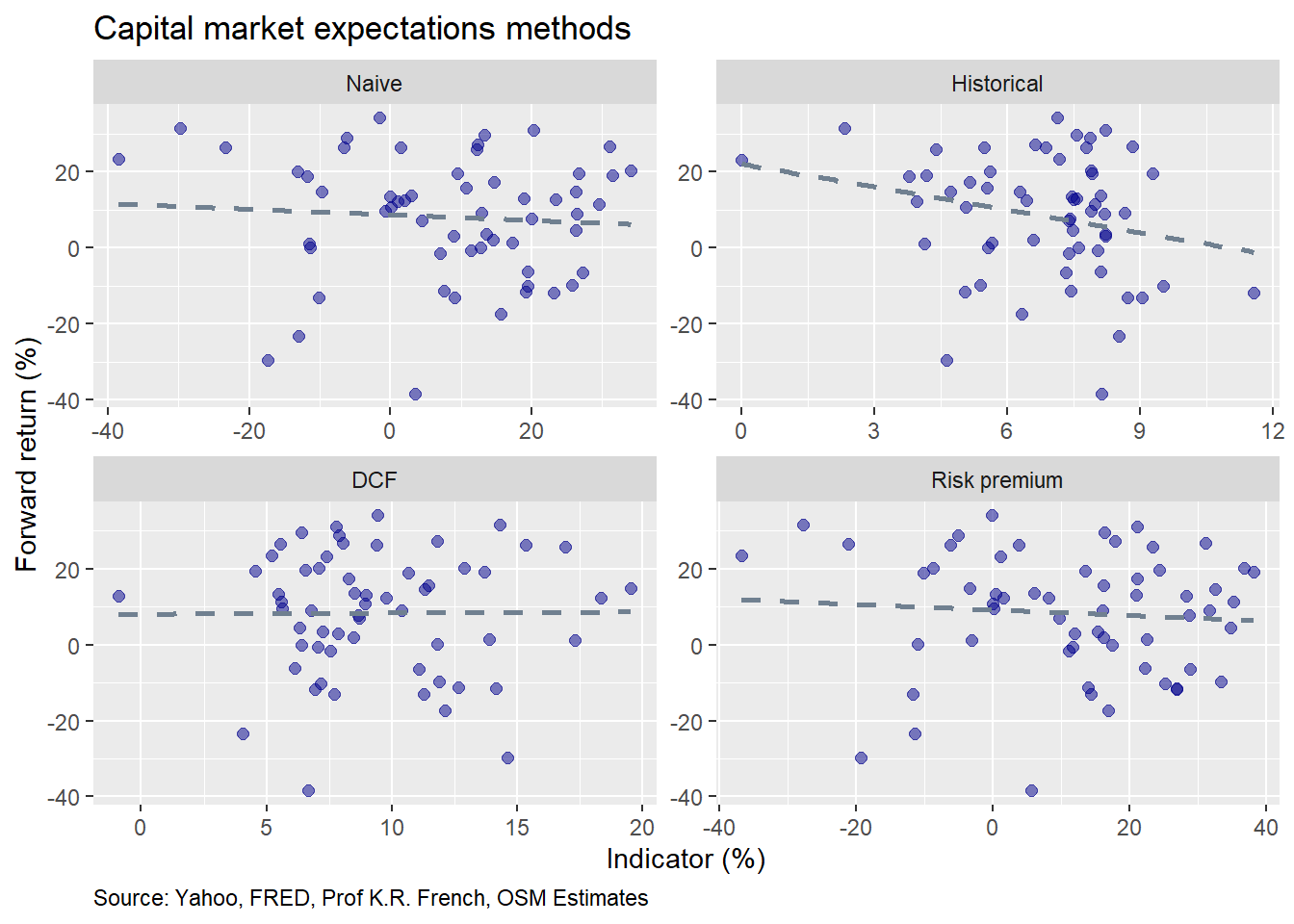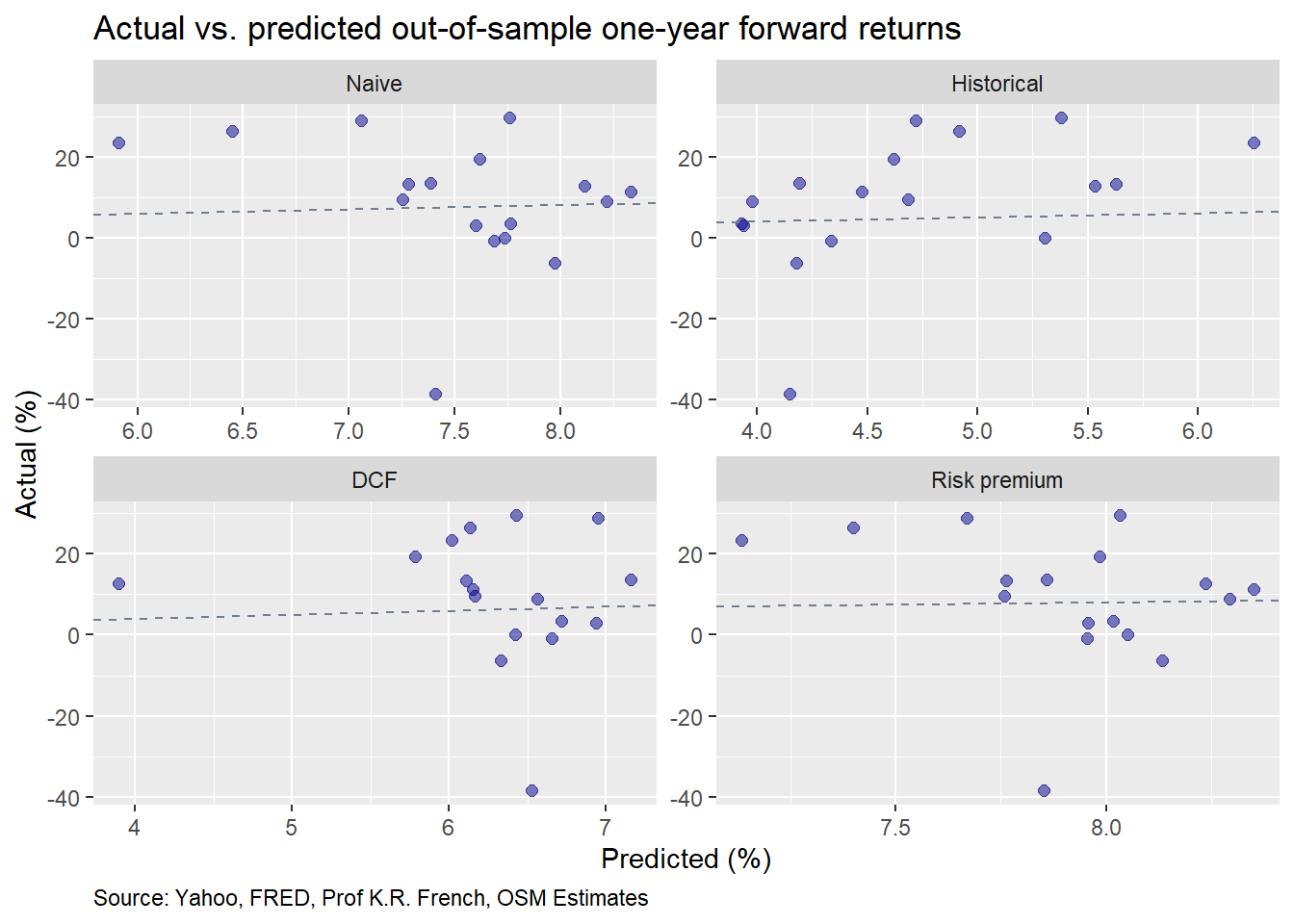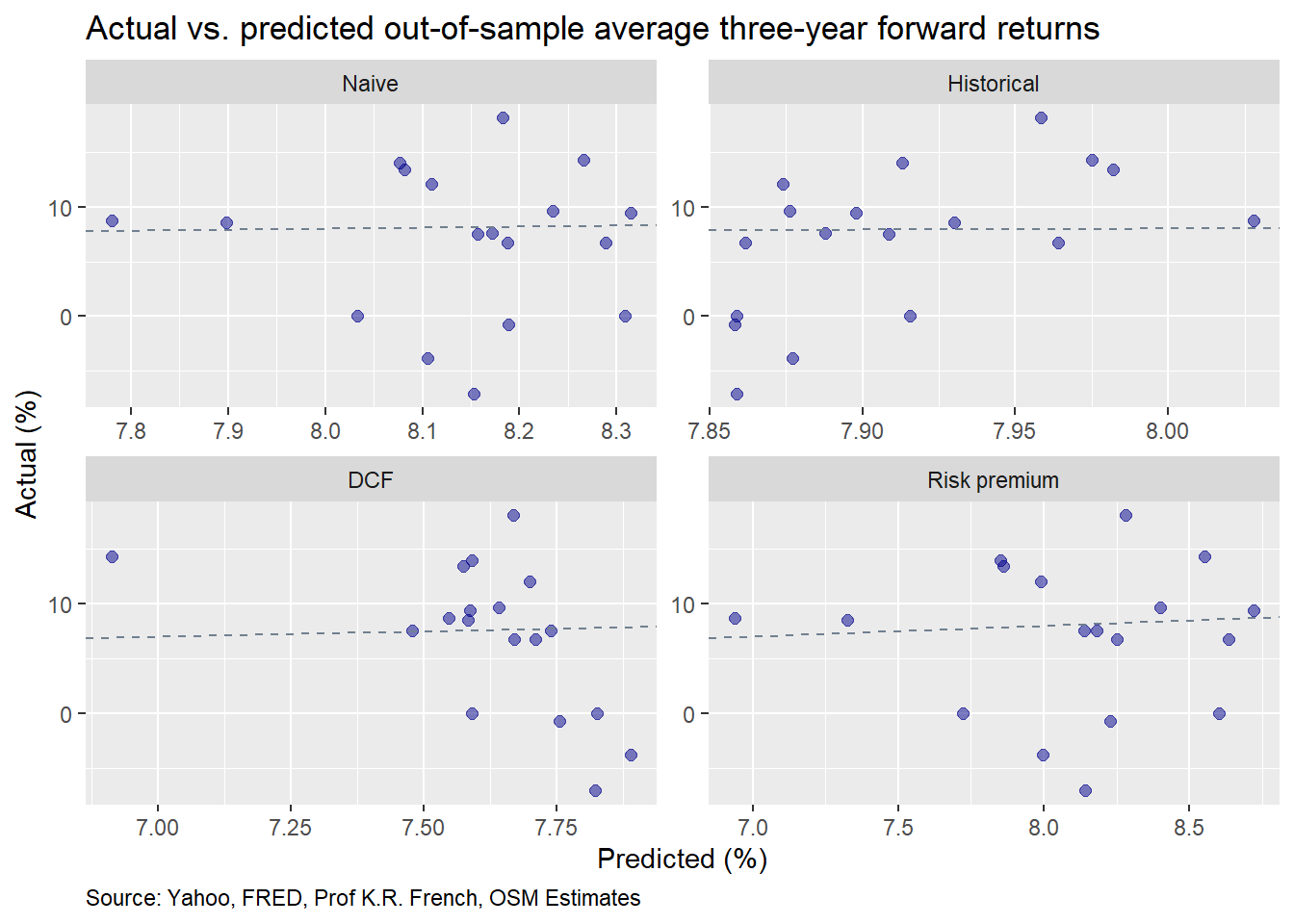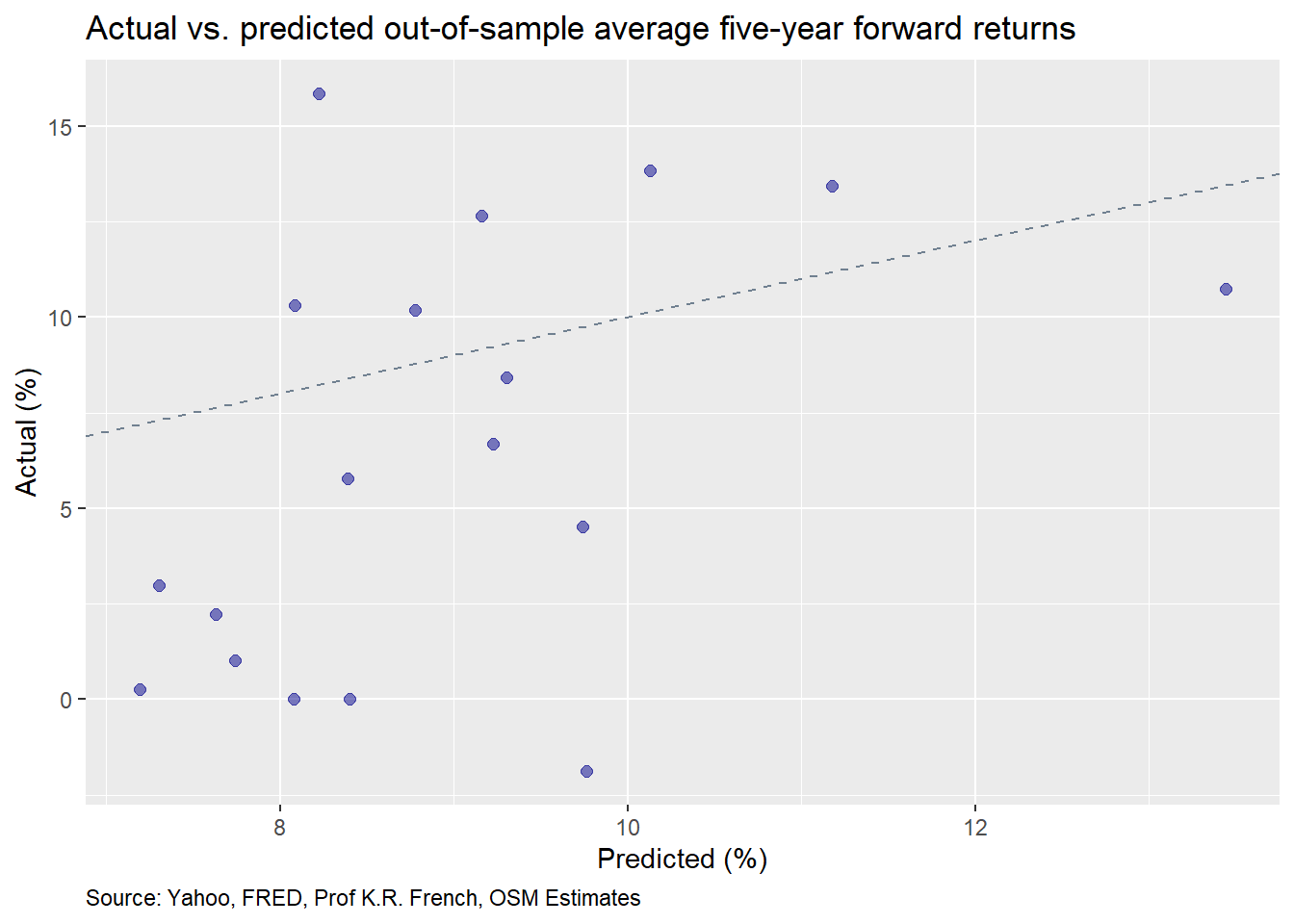Over the past few weeks, we’ve examined the three major methods used to set return expectations as part of the portfolio allocation process. Those methods were historical averages, discounted cash flow models, and risk premia models. Today, we’ll bring all these models together to compare and contrast their accuracy.

Before we make these comparisons, we want to remind readers that we’re now including a python version of the code we use to produce our analyses and graphs. Let us know if you find this useful. Second, let’s remind ourselves why we’re analyzing these return expectations methods in the first place, Recall, to construct a satisfactory portfolio one needs estimates of both return and risk for the various assets that merit inclusion. Once you have those estimates, you can use them to combine assets in numerous ways to generate a bunch of portfolios with different risk-return combinations. Hopefully, some of these combinations will fit your required risk/return criteria.

Our project, then, has been to introduce these different methods so that we can ultimately decide which one seems the most useful to help make portfolio allocation decisions. Importantly, we should define the criteria for how we’re going to assess these methods. In the prior posts, we examined the methods’ ability to forecast future returns using the error rate as the main judge. When we actually get down to deciding on our asset allocation, we’re not going to be as concerned with having the best estimate of future returns. Rather, we’re looking to identify portfolios that offer the best risk-adjusted returns based on our estimates. This might seem like too fine of a point. But it is important to note that, assuming we’re trying to build a reasonably diversified portfolio, the accuracy of any individual return estimate will be dampened by the number of assets in the portfolio. Thus we don’t need “perfect” estimates to create a “good” portfolio.

In any case, here’s the plan for this post. We’ll start off exploring the different methods graphically. Then we’ll run the basic models over different time frames. Then we’ll consider whether pooling the predictions can improve results.

Before we begin, it’s important to flag some of the methodological hurdles this analysis faces. We’re using data for the S&P 500, US nominal GDP, and the market risk premium, as published by Dartmouth professor Kenneth R. French. Additionally, the discounted cash flow (DCF) model we analyzed in a prior post, dispensed with the dividend yield and assumed the required return would be roughly equivalent to the growth rate of nominal GDP. This was clearly a short cut and could be considered sloppy.1 For this post we fix that so that the risk premium is calculated via a simplified DCF.

There are also data compilation issues: the frequencies don’t all align. S&P data are daily, GDP data are quarterly, and market risk premium data are estimated annually or monthly (but with a much shorter time frame). Hence, we decided to go with annual data since that would provide us with the longest overlapping series, starting in 1960. Such frequency matches the shortest time horizon for most longer term oriented professional and individual investors. Many, however, use shorter time spans.

Finally, let’s summarize how each method is constructed:

• Naive: This is our benchmark. It essentially takes last year’s return as the predictor for next year’s.

• Historical: This is the cumulative return for the data series. Hence, as we roll forward in time any single year’s influence on the prediction decreases at a decelerating rate.

• DCF: This is the expected return calculated using the Gordon Growth model, based on the S&P’s dividend yield, and nominal GDP growth, as a proxy for the growth rate. GDP growth is calculated using the twelve months ending in the third quarter. Recall, final GDP data are delayed by a quarter, so anyone conducting the analysis in real time would only have third quarter data available at year-end.

Housekeeping and introductions over, let’s begin. We’ll first compile the data and then graph the end-of-year “signal” on the x-axis and the following year’s return for the S&P 500 on the y-axis.A few points to notice. The Naive, DCF, and Risk premium methods show almost no relationship between the explanatory variable and next year’s return. Historical averages suggest a negative relationship with forward returns consistent with the mean reversion present in the series.2.

We could run a few more graphs, but let’s jump into the machine learning. We’ll train the models on 70% of the data, reserving the remainder for the test set. We’ll then show the outputs of the models with actual vs predicted graphs on the out-of-sample data. We’ll follow that with accuracy tables based on root mean-squared error (RMSE) and the error scaled by average of the actual results.

Here’s the first model in which we regress the one-year forward return on the S&P against the return expectation generated by the respective method. We include a 45o line to highlight error.Both the Naive and DCF methods sport a fair amount of clustering. The Historical and Risk premium methods are better distributed. Note that even though we included a 45o line it doesn’t appear that way, due to the graphing algorithm, which does not scale each axis equally. Of course, if it did, we wouldn’t be able to make out the dispersion in the predicted values given the spread in the actuals. Another problem is 2008, the global financial crisis, which posted a loss close to 40%. It looks like an outlier, but needs to be there given the character of financial returns. Let’s look at the accuracy scores.

Table 1: Machine learning accuracy one-year forward returns
Model Train: RMSE Train: RMSE scaled Test: RMSE Test: RMSE scaled
Naive 0.16 2.03 0.16 1.72
Historical 0.15 1.94 0.16 1.73
DCF 0.16 2.02 0.16 1.73
Risk premium 0.16 2.02 0.16 1.71

The RMSE for both the training and test sets are very similar both within and across groups. The scaled error declines from training to test sets for all methods. This is because the average returns in the test set are about 17% higher than the training set. So the improvement is data, not model, related. Whatever the case, no method stands out as better on the test set.

Now, we’ll look at how well each of the methods predicts average returns over the next three years. The averaging should smooth out some of the volatility and produce slightly better forecasts. First, up is the actual vs. predicted graph.In general, the clustering improves, except for the DCF method. In most cases, the models’ predicted values over or undershoot the actual values equally. Let’s check out the accuracy scores.

Table 2: Machine learning accuracy average three-year forward returns
Model Train: RMSE Train: RMSE scaled Test: RMSE Test: RMSE scaled
Naive 0.09 1.09 0.07 0.97
Historical 0.09 1.09 0.07 0.96
DCF 0.09 1.09 0.07 0.97
Risk premium 0.09 1.09 0.07 0.97

As expected, the accuracy is better on both the absolute and scaled RMSE. There appears little difference among the methods as well.

Now, we’ll look at each method against the average five-year forward return to see if that improves accuracy further. Here’s the actual vs. predicted graph.Clustering improves modestly. Outliers persist. In this instance, the historical method seems to show the most balanced dispersion around the 45o line. Note that for the Risk premium method, the model appears to over estimate returns slightly, as shown by more points below the line than above. Let’s check on the accuracy.

Table 3: Machine learning accuracy average five-year forward returns
Model Train: RMSE Train: RMSE scaled Test: RMSE Test: RMSE scaled
Naive 0.07 0.81 0.06 0.90
Historical 0.07 0.80 0.05 0.83
DCF 0.07 0.82 0.06 0.88
Risk premium 0.07 0.82 0.06 0.89

The absolute and scaled RMSE improved again. The Historical method saw the biggest improvement, which should be expected if there is some latent mean reversion in the returns. Importantly, all the three methods show better scaled accuracy than the Naive benchmark; though, the Risk Premium method isn’t that dramatic.

Which method should we choose? Part of that depends on what our time horizon is. If we want to rebalance the portfolio every year based on revised expectations, then one might prefer the Risk premium method. But it’s accuracy isn’t so much better that it’s a clear winner.

If we’ re looking at longer time frames, then the Historical or DCF methods appear to enjoy better accuracy, with the Historical being meaningfully better on the five-year horizon. It’s also the easiest to calculate. But the warning is that there is no guarantee that the future will look at all like the past.

If one method is good, shouldn’t three be better? We’ll now examine how a multivariate model of all three methods performs and then compare it to an ensemble approach where we average the predictions of each method. Before we do that we should check the correlations among the different explanatory variables to avoid multi-collinearity. In other words, if the different methods are highly correlated, using them to model or predict the same thing will generate results that seem more significant than they actually are.

Table 4: Explanatory variable correlations
Historical 1.00 -0.56 0.36
DCF -0.56 1.00 -0.21

As the table shows, the correlations are relatively low and/or negative, so we should be safe. While we’ve run through all the time periods offline, we’ll show the average five-year forward return only for the sake of brevity. It also performs the best relative to the other methods. The usual actual vs. predicted graph is below.We now compare the multivariate accuracy to the average of the univariate methods plus the Naive method for the average five-year forward return. Note that for univariate average, we’re averaging the prediction of each method and then comparing that to the actual.

Table 5: Machine learning accuracy average five-year forward returns
Model Test: RMSE Test: RMSE scaled
Naive 0.06 0.90
Univariate 0.06 0.86
Multivariate 0.06 0.86

The multivariate method performs about the same as averaging the predictions generated by each of the univariate methods. Both perform better than the Naive method on a scaled basis. For many folks, this is probably as far as one wants or needs to go. The ensemble methods perform better than all but the Historical method. And both univariate and multivariate outperform the Naive method. Since there’s little difference between the univariate average and multivariate model, using the multivariate is probably more efficient since it cuts down on calculations (thanks for R).

What have we learned? No particular method tends to dominate the others on all time frames. In general, as we extend the time frame, prediction accuracy improves for all methods, including the Naive. Of course, all this relates only to one proxy of one asset class: the S&P 500 for equities. To build a diversified portfolio one would want to conduct the same analyses for bonds, and real assets too.

That prediction accuracy improved with longer time frames seems a key insight to us. It wasn’t better models that improved the results, it was a more nuanced understanding of the data’s structure. Of course, we didn’t employ more sophisticated models like random forests or neural networks, nor did we experiment with any sort of feature selection. These avenues might prove fruitful or dead-ends. Whichever the case, fleshing them out would require additional posts.

Our next step, will be extend these methods across the different asset classes to build a diversified portfolio. Alternatively, we could explore more sophisticated statistical learning techniques before moving on. If you have a view on which you’d prefer, send us a message at the email below. Until next time the R code, followed by the python code for this post is below.

# Built using R 3.6.2

suppressPackageStartupMessages({
library(tidyquant)
library(tidyverse)
library(httr)
})

## Note this is a bit messy as I already had the files from the different posts, which I loaded
## and then joined. But it's not very helpful to see df <- readRDS("clean_file.rds"). So I show how I
## pulled the data for the various files from each of the previous posts. Probably a cleaner way to do
## this, but I hope you get the picture.

## Fama-French factors
url <- "http://mba.tuck.dartmouth.edu/pages/faculty/ken.french/ftp/F-F_Research_Data_Factors_CSV.zip"
GET(url, write_disk(tf1 <- tempfile(fileext = ".zip")))

erp <- erp %>%
slice(1130:(nrow(erp)-1)) %>%
rename("year" = X1,
"mkt" = Mkt-RF,
"rf" = RF)

## GDP data to align with S&P 500
sp <- getSymbols("^GSPC", src = "yahoo", from = "1950-01-01", to = "2020-01-01",
auto.assign = FALSE) %>%
colnames<-("sp")

sp_qtr <- to.quarterly(sp, indexAt = "lastof", OHLC = FALSE)
gdp <- getSymbols("GDP", src = "FRED", from = "1950-01-01", to = "2020-01-01",
auto.assign = FALSE) %>%
colnames<-("gdp")
qtr <- index(to.quarterly(sp["1950/2019"], indexAt = "lastof", OHLC = FALSE))
gdp_eop <- xts(coredata(gdp["1950/2019"]), order.by = qtr)

gdp_growth <- gdp %>%
mutate(year = year(date),
gdp_lag = lag(gdp_ret)) %>%
group_by(year) %>%
mutate(gdp_yr = sum(gdp_lag)) %>%
distinct(year, gdp_yr)

GET(url1, write_disk(tf <- tempfile(fileext = ".xls")))
ipr <- irp[-61,]

df <- irp %>%
left_join(gdp_growth, by = "year") %>%
left_join(erp, by = "year") %>%
select(-c(t_bill, bond_bill, erp_ddm, erp_fcfes, SMB, HML)) %>%
mutate_at(vars(mkt, rf), function(x) x/100) %>%
mutate(cum_ret = cummean(ifelse(is.na(sp_ret),0,sp_ret)),
req_ret = div_yld*(1+gdp_yr)+gdp_yr,
rp = mkt + rf,
sp_mu3 = runMean(ifelse(is.na(sp_fwd3),0,sp_fwd3), 3),
sp_mu5 = runMean(ifelse(is.na(sp_fwd5),0,sp_fwd5), 5))

## Graph previous vs next
df %>%
select(year, sp_ret, cum_ret, req_ret, rp, sp_fwd) %>%
gather(key,value, -c(year, sp_fwd)) %>%
mutate(key = factor(key, levels = c("sp_ret", "cum_ret", "req_ret", "rp"))) %>%
ggplot(aes(value*100, sp_fwd*100))+
geom_point(color = "darkblue", size = 2, alpha = 0.5) +
geom_smooth(method = "lm", se = FALSE, linetype="dashed", color="slategrey") +
facet_wrap(~key, scales = "free", labeller = as_labeller(c(sp_ret = "Naive",
cum_ret = "Historical",
req_ret = "DCF",
labs(x = "Indicator (%)",
y = "Forward return (%)",
title = "Capital market expectations methods",
caption = "Source: Yahoo, FRED, Prof K.R. French, OSM Estimates") +
theme(plot.caption = element_text(hjust = 0))

### Machine learning
## Create and run machine learning function and rmse table output function
ml_func <- function(train_set, test_set, x_var1, y_var){
form <- as.formula(paste(y_var, " ~ ", x_var1))
mod <- lm(form, train_set)

act_train <- train_set[,y_var] %>% unlist() %>% as.numeric()
act_test <- test_set[,y_var] %>% unlist() %>% as.numeric()

pred_train <- predict(mod, train_set)

rmse_train <- sqrt(mean((pred_train - act_train)^2, na.rm = TRUE))
rmse_train_scaled <- rmse_train/mean(act_train,na.rm = TRUE)

pred_test <- predict(mod, test_set)
rmse_test <- sqrt(mean((pred_test - act_test)^2, na.rm = TRUE))
rmse_test_scaled <- rmse_test/mean(act_test ,na.rm = TRUE)

out = list(coefs = coef(mod),
pred_train=pred_train,
rmse_train=rmse_train,
rmse_train_scaled=rmse_train_scaled,
pred_test=pred_test,
rmse_test=rmse_test,
rmse_test_scaled=rmse_test_scaled)

out

}

rmse_table <- function(naive, hist, dcf, rp){
data.frame(Model = c("Naive", "Historical", "DCF", "Risk premium"),
train_rmse = c(naive$rmse_train, hist$rmse_train, dcf$rmse_train, rp$rmse_train),
train_rmse_scaled =  c(naive$rmse_train_scaled, hist$rmse_train_scaled,
dcf$rmse_train_scaled, rp$rmse_train_scaled),
test_rmse = c(naive$rmse_test, hist$rmse_test, dcf$rmse_test, rp$rmse_test),
test_rmse_scaled = c(naive$rmse_test_scaled, hist$rmse_test_scaled,
dcf$rmse_test_scaled, rp$rmse_test_scaled)) %>%
mutate_at(vars(-Model), function(x) round(x,2)) %>%
rename("Train: RMSE" = train_rmse,
"Train: RMSE scaled" = train_rmse_scaled,
"Test: RMSE" = test_rmse,
"Test: RMSE scaled" = test_rmse_scaled) %>%
knitr::kable(caption = "Machine learning accuracy")

}

ml_output_graf <- function(df, y_var = "sp_fwd", naive, hist, dcf, rp){
df[,y_var] %>%
colnames<-("actual") %>%
mutate(naive = naive$pred_test, hist = hist$pred_test,
dcf = dcf$pred_test, rp = rp$pred_test) %>%
select(actual, naive, hist, dcf, rp) %>%
gather(key, value, -actual) %>%
mutate(key = factor(key, levels = c("naive", "hist", "dcf", "rp"))) %>%
ggplot(aes(value*100, actual*100)) +
geom_point(color = "darkblue", size = 2, alpha = 0.5) +
geom_abline(linetype="dashed", color="slategrey") +
facet_wrap(~key, scales = "free", labeller = as_labeller(c(naive = "Naive",
hist = "Historical",
dcf = "DCF",
labs(x = "Predicted (%)",
y = "Actual (%)",
title = "Actual vs. predicted out of sample",
caption = "Source: Yahoo, FRED, Prof K.R. French, OSM Estimates") +
theme(plot.caption = element_text(hjust = 0))
}

## Split data
split <- round(nrow(df)*.7,0)
train <- df[1:split,]
test <- df[(split+1):nrow(df),]

## Split data
split <- round(nrow(df)*.7,0)
train <- df[1:split,]
test <- df[(split+1):nrow(irp_growth),]

## Run one yar model
naive <- ml_func(train, test, "sp_ret", "sp_fwd")
hist <- ml_func(train,test, "cum_ret", "sp_fwd")
dcf <- ml_func(train,test, "req_ret", "sp_fwd")
rp <- ml_func(train,test, "rp", "sp_fwd")

## Show graph
ml_output_graf(test, y_var="sp_fwd", naive, hist, dcf, rp)

## Print table
rmse_table(naive, hist, dcf, rp)

## Run 3 year average
naive <- ml_func(train, test, "sp_ret", "sp_mu3")
hist <- ml_func(train,test, "cum_ret", "sp_mu3")
dcf <- ml_func(train,test, "req_ret", "sp_mu3")
rp <- ml_func(train,test, "rp", "sp_mu3")

## Show graph
ml_output_graf(test, y_var = "sp_mu3", naive, hist, dcf, rp)

## Print table
rmse_table(naive, hist, dcf, rp)

## Run 5 year average
naive <- ml_func(train, test, "sp_ret", "sp_mu5")
hist <- ml_func(train,test, "cum_ret", "sp_mu5")
dcf <- ml_func(train,test, "gdp_yr", "sp_mu5")
rp <- ml_func(train,test, "erp_fcfe", "sp_mu5")

## Show graph
ml_output_graf(test, "sp_mu5", naive, hist, dcf, rp)

## Print table
rmse_table(naive, hist, dcf, rp)

## Correlation table
train %>%
select(cum_ret, req_ret, rp) %>%
rename("Historical" = cum_ret,
"DCF" = req_ret,
cor(.) %>%
round(.,2) %>%
knitr::kable(caption= "Correlations of explanatory variables")

## Run multivariate regresssion
combo_mod <- lm(sp_mu5 ~ cum_ret + req_ret + rp, train)
preds <- predict(combo_mod, test)

rmse_comb <- sqrt(mean((preds - test$sp_mu5)^2, na.rm = TRUE)) rmse_comb_scaled <- rmse_comb/mean(test$sp_mu5, na.rm = TRUE)

## Graph of multivariate actual vs predicted
ggplot() +
geom_point(aes(preds*100, test$sp_mu5*100), color = "darkblue", size = 2, alpha = 0.5) + geom_abline(linetype="dashed", color="slategrey") + labs(x = "Predicted (%)", y = "Actual (%)", title = "Actual vs. predicted out of sample", caption = "Source: Yahoo, FRED, Prof K.R. French, OSM Estimates") + theme(plot.caption = element_text(hjust = 0)) # Caclualate average of predictions and accuracy preds_mean <- rowMeans(cbind(hist$pred_test, dcf$pred_test, rp$pred_test))
rmse_mean <- sqrt(mean((preds_mean - test$sp_mu5)^2, na.rm = TRUE)) rmse_mean_scaled <- rmse_comb/mean(test$sp_mu5, na.rm = TRUE)

# Print table of the results
data.frame(Model = c("Naive", "Univariate", "Multivariate"),
test_rmse = c(naive$rmse_test, rmse_mean, rmse_comb), test_rmse_scaled = c(naive$rmse_test_scaled,
rmse_mean_scaled,
rmse_comb_scaled)) %>%
mutate_at(vars(-Model), function(x) round(x,2)) %>%
rename("Test: RMSE" = test_rmse,
"Test: RMSE scaled" = test_rmse_scaled) %>%
knitr::kable(caption = "Machine learning accuracy")

And here’s the python code for pythonistas:

# Built using python 3.7

# NOTE: Python results don't always match what I get in R. Sometimes I think that might be the way
# scikit-learn handles NaNs, but I'm not entirely sure. Let me know if you get something wildly
# different.

import pandas as pd
import numpy as np
import matplotlib.pyplot as plt
import seaborn as sns
%matplotlib inline

plt.style.use('ggplot')
sns.set()

# Note the R code shows how I wrangled the data. Due to time constraints, I saved that as a csv
# and loaded in the python enviroment. I hope to come back and show the pythonic way to get the data
# at some point.

#### Plot different methods
fig = plt.figure(figsize=(14,10))

sns.regplot(df.sp_ret, df.sp_fwd, ax = a1, color="darkblue", ci=None)
sns.regplot(df.cum_ret, df.sp_fwd, ax = a2,color="darkblue", ci=None)
sns.regplot(df.req_ret, df.sp_fwd, ax = a3,color="darkblue", ci=None)
sns.regplot(df.rp, df.sp_fwd, ax = a4,color="darkblue", ci=None)

axs = [a1, a2, a3, a4]
titles = ["Naive", "Historical", "DCF", "Risk premium"]
for i in range(4):
axs[i].set_title(titles[i], fontsize=14)

if i < 2:
axs[i].set_xlabel("")
else:
axs[i].set_xlabel("Actual")

if i % 2 != 0:
axs[i].set_ylabel("")
else:
axs[i].set_ylabel("Return")

fig.suptitle("Return expectations methods")
plt.figtext(0,0, "Source: Prof. A. Damodaran, NYU")
# plt.tight_layout()
plt.show()

#### Machine learning
x = df[['sp_ret', 'cum_ret', 'req_ret', 'rp']]
y = df[['sp_fwd', 'sp_mu3', 'sp_mu5']]

#### Create machine learning function
def ml_func(x, y, x_var, y_var):
from sklearn.linear_model import LinearRegression

rows = int(len(x)*.7)

x_train = x.loc[1:rows, x_var].values.reshape(-1,1)
y_train = y.loc[1:rows, y_var].values.reshape(-1,1)
x_test = x.loc[rows:, x_var].values.reshape(-1,1)
y_test = y.loc[rows:, y_var].values.reshape(-1,1)

mod = LinearRegression()
mod.fit(x_train, y_train)

pred_train = mod.predict(x_train)
rmse_train = np.sqrt(np.nanmean((pred_train - y_train)**2))
rmse_train_scaled = rmse_train/np.nanmean(y_train)

pred_test = mod.predict(x_test)
rmse_test = np.sqrt(np.nanmean((pred_test - y_test)**2))
rmse_test_scaled = rmse_test/np.nanmean(y_test)

return pred_test, y_test, rmse_train, rmse_train_scaled, rmse_test, rmse_test_scaled

#### Run 1-year model
naive = ml_func(x, y, 'sp_ret', 'sp_fwd')
hist = ml_func(x, y, 'cum_ret', 'sp_fwd')
dcf = ml_func(x, y, 'req_ret', 'sp_fwd')
rp = ml_func(x, y, 'rp', 'sp_fwd')

#### Create graphing function
def plot_ml(naive, hist, dcf, rp):
fig = plt.figure(figsize=(14,10))

a1.scatter(naive*100, naive*100, color="darkblue", s=100, alpha=0.5)
xn = naive*100
yn = xn
a1.plot(xn,yn, linestyle=":", color='grey')

a2.scatter(hist*100, hist*100, color="darkblue", s=100, alpha=0.5)
xh = hist*100
yh = xh
a2.plot(xh,yh, linestyle=":",color='grey')

a3.scatter(dcf*100, dcf*100, color="darkblue", s=100, alpha=0.5)
xd = dcf*100
yd = xd
a3.plot(xd,yd, linestyle=":",color='grey')

a4.scatter(rp*100, rp*100, color="darkblue", s=100, alpha=0.5)
xr = rp*100
yr = xr
a4.plot(xr,yr, linestyle=":",color='grey')

axs = [a1, a2, a3, a4]
titles = ["Naive", "Historical", "DCF", "Risk premium"]
for i in range(4):
axs[i].set_title(titles[i], fontsize=14)

if i < 2:
axs[i].set_xlabel("")
else:
axs[i].set_xlabel("Predicted")

if i % 2 != 0:
axs[i].set_ylabel("")
else:
axs[i].set_ylabel("Actual")

fig.suptitle("Machine learning methods")
plt.figtext(0,0, "Source: Yahoo, FRED, Prof. A. Damodaran, NYU, OSM estimates")
# plt.tight_layout()
plt.show()

#### Graph 1-year model
plot_ml(naive, hist, dcf, rp)

#### Create ml table
def ml_table(naive, hist, dcf, rp):
table =  pd.DataFrame({'Model': ["Naive", "Historical", "DCF", "Risk premium"],
'Train RMSE': [naive, hist, dcf, rp],
"Train: RMSE scaled" :  [naive, hist, dcf, rp],
"Test: RMSE": [naive, hist, dcf, rp],
"Test: RMSE scaled": [naive, hist, dcf, rp]})

return round(table,2)

#### Print 1-year model table
ml_table(naive, hist, dcf, rp)

#### Run Average 3-year forward models
# Need to index at 2 since scikit-learn doesn't seem to handle NaNs like R handles NAs
naive = ml_func(x[2:], y[2:], "sp_ret", "sp_mu3")
hist = ml_func(x[2:], y[2:], "cum_ret", "sp_mu3")
dcf = ml_func(x[2:], y[2:], "req_ret", "sp_mu3")
rp = ml_func(x[2:] ,y[2:], "rp", "sp_mu3")

##### Plot actual vs predicted
plot_ml(naive, hist, dcf, rp)

##### Print accuracy tables
ml_table(naive, hist, dcf, rp)

#### Run averae five-year forward models
# Need to index at 4 since scikit-learn doesn't seem to handle NaNs like R handles NAs
naive = ml_func(x[4:], y[4:], "sp_ret", "sp_mu5")
hist = ml_func(x[4:], y[4:], "cum_ret", "sp_mu5")
dcf = ml_func(x[4:], y[4:], "req_ret", "sp_mu5")
rp = ml_func(x[4:] ,y[4:], "rp", "sp_mu5")

plot_ml(naive, hist, dcf, rp)
ml_table(naive, hist, dcf, rp)

#### Correlation analysis

rows = int(len(x)*.7)
corr_df = x.loc[1:rows,['cum_ret', 'req_ret', 'rp']]
corr_df.columns = ['Historical', 'DCF', 'Risk premium']
corr_df.corr().round(2)

#### Multivariate model
from sklearn.linear_model import LinearRegression

rows = int(len(x)*.7)

x_train = x.loc[4:rows, ['cum_ret', 'req_ret', 'rp']].values.reshape(-1,3)
y_train = y.loc[4:rows, 'sp_mu5'].values.reshape(-1,1)
x_test = x.loc[rows:, ['cum_ret', 'req_ret', 'rp']].values.reshape(-1,3)
y_test = y.loc[rows:, 'sp_mu5'].values.reshape(-1,1)

mod = LinearRegression()
mod.fit(x_train, y_train)

# pred_train = mod.predict(x_train)
# rmse_train = np.sqrt(np.nanmean((pred_train - y_train)**2))
# rmse_train_scaled = rmse_train/np.nanmean(y_train)

pred_test = mod.predict(x_test)
rmse_test = np.sqrt(np.nanmean((pred_test - y_test)**2))
rmse_test_scaled = rmse_test/np.nanmean(y_test)

# return pred_test, y_test, rmse_train, rmse_train_scaled, rmse_test, rmse_test_scaled

plt.figure(figsize=(12,6))
plt.scatter(pred_test*100, y_test*100, color="darkblue", s=100, alpha=0.5)
xs = pred_test*100
ys = xs
plt.plot(xs,ys, linestyle=":",color='grey')
plt.xlabel("Predicted return (%)")
plt.ylabel("Actual return(%)")
plt.title("Actual vs. predicted results for machine learning model")
plt.show()

preds_mean = pd.DataFrame({'hist':hist.reshape(21), 'dcf':dcf.reshape(21), 'rp':rp.reshape(21)}, index=np.arange(0,21)).mean(axis=1)
rmse_mean = np.sqrt(np.mean((preds_mean.values - hist)**2))
rmse_mean_scaled = rmse_mean/np.mean(hist)

multi_mods = pd.DataFrame({'Model':["Naive", "Univariate", "Multivariate"],
'Test: RMSE': [naive,rmse_mean, rmse_test],
'Test: RMSE scale': [naive, rmse_mean_scaled, rmse_test_scaled]})
multi_mods.round(2)

1. The results are actually not that different. But we don’t want to get buried in too many details.

2. All covariance stationary data time series have a finite mean reverting level. Who knows what that means, but we’ve always wanted to write that!